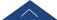﻿ 20180528 冯兆生-Bounded Wave Solutions to the KdV-Burgers-Kuramoto equation-湖南大学数学与计量经济学院

# 20180528 冯兆生-Bounded Wave Solutions to the KdV-Burgers-Kuramoto equation

In this talk, we consider the KdV-Burgers-Kuramoto equation, a partial differential equation that occupies a prominent position in describing some physical processes in motion of turbulence and other unstable process systems. Equivalence transformations are applied for exploring the principal Lie algebra. By means of the associated equivalence algebra we convert partial differential equations into a system of ordinary differential equations from which we can derive some explicit and implicit solutions.